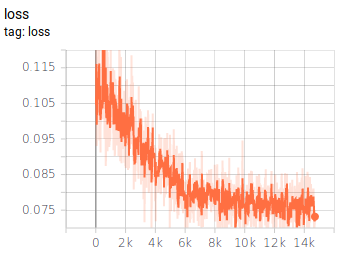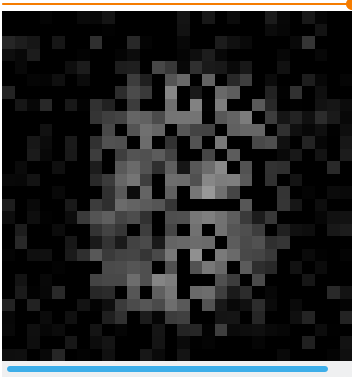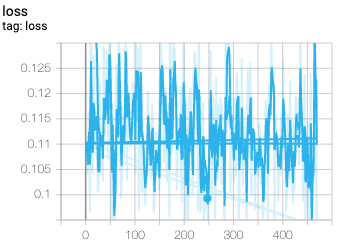Skip to content
{{ message }}

Instantly share code, notes, and snippets.

# AFAgarap/autoencoder-full.py

Last active Apr 23, 2021
TensorFlow 2.0 implementation for a vanilla autoencoder. Link to tutorial: https://medium.com/@abien.agarap/implementing-an-autoencoder-in-tensorflow-2-0-5e86126e9f7
 """TensorFlow 2.0 implementation of vanilla Autoencoder.""" import numpy as np import tensorflow as tf __author__ = "Abien Fred Agarap" np.random.seed(1) tf.random.set_seed(1) batch_size = 128 epochs = 10 learning_rate = 1e-2 intermediate_dim = 64 original_dim = 784 (training_features, _), _ = tf.keras.datasets.mnist.load_data() training_features = training_features / np.max(training_features) training_features = training_features.reshape(training_features.shape, training_features.shape * training_features.shape) training_features = training_features.astype('float32') training_dataset = tf.data.Dataset.from_tensor_slices(training_features) training_dataset = training_dataset.batch(batch_size) training_dataset = training_dataset.shuffle(training_features.shape) training_dataset = training_dataset.prefetch(batch_size * 4) class Encoder(tf.keras.layers.Layer): def __init__(self, intermediate_dim): super(Encoder, self).__init__() self.hidden_layer = tf.keras.layers.Dense( units=intermediate_dim, activation=tf.nn.relu, kernel_initializer='he_uniform' ) self.output_layer = tf.keras.layers.Dense( units=intermediate_dim, activation=tf.nn.sigmoid ) def call(self, input_features): activation = self.hidden_layer(input_features) return self.output_layer(activation) class Decoder(tf.keras.layers.Layer): def __init__(self, intermediate_dim, original_dim): super(Decoder, self).__init__() self.hidden_layer = tf.keras.layers.Dense( units=intermediate_dim, activation=tf.nn.relu, kernel_initializer='he_uniform' ) self.output_layer = tf.keras.layers.Dense( units=original_dim, activation=tf.nn.sigmoid ) def call(self, code): activation = self.hidden_layer(code) return self.output_layer(activation) class Autoencoder(tf.keras.Model): def __init__(self, intermediate_dim, original_dim): super(Autoencoder, self).__init__() self.encoder = Encoder(intermediate_dim=intermediate_dim) self.decoder = Decoder( intermediate_dim=intermediate_dim, original_dim=original_dim ) def call(self, input_features): code = self.encoder(input_features) reconstructed = self.decoder(code) return reconstructed autoencoder = Autoencoder( intermediate_dim=intermediate_dim, original_dim=original_dim ) opt = tf.optimizers.Adam(learning_rate=learning_rate) def loss(model, original): reconstruction_error = tf.reduce_mean(tf.square(tf.subtract(model(original), original))) return reconstruction_error def train(loss, model, opt, original): with tf.GradientTape() as tape: gradients = tape.gradient(loss(model, original), model.trainable_variables) gradient_variables = zip(gradients, model.trainable_variables) opt.apply_gradients(gradient_variables) writer = tf.summary.create_file_writer('tmp') with writer.as_default(): with tf.summary.record_if(True): for epoch in range(epochs): for step, batch_features in enumerate(training_dataset): train(loss, autoencoder, opt, batch_features) loss_values = loss(autoencoder, batch_features) original = tf.reshape(batch_features, (batch_features.shape, 28, 28, 1)) reconstructed = tf.reshape(autoencoder(tf.constant(batch_features)), (batch_features.shape, 28, 28, 1)) tf.summary.scalar('loss', loss_values, step=step) tf.summary.image('original', original, max_outputs=10, step=step) tf.summary.image('reconstructed', reconstructed, max_outputs=10, step=step)

###lorenzo-rovigatti commented Aug 8, 2019 • edited

 Hey, thanks a bunch for this gist. I am quite new to TF (and machine/deep learning in general) and this is the kind of stuff that is really helping me. However, I cannot seem to make it work. This is the loss function I get (after more than 10 epochs):It seems to plateau after an initial descent, and the reconstructed pictures all look like this one:Differently from your tutorial, I am using TF 2.0.0-beta1. Is there anything that has between the alpha and the beta versions and could have broken this gist? Edit: it looks like using an Adam optimiser rather than the SGD solves this issue.

###AFAgarap commented Aug 8, 2019

 Thanks, @lorenzo-rovigatti. Sorry, I wasn't able to respond sooner. Yes, I also used Adam in my experiment with this autoencoder in TF 2.0.0-beta1.

###lorenzo-rovigatti commented Aug 8, 2019

 OK good to know, thanks!

###eoehri commented Dec 21, 2019

 Hi, thanks for sharing this. As you suggested in your Medium article I tried to implement a CNN architecture but something isn't working properly. My restructured images are all black. And this is the loss I get:The code: click here Can you help me? What am I doing wrong? Thanks for your appreciated help!
to join this conversation on GitHub. Already have an account? Sign in to comment